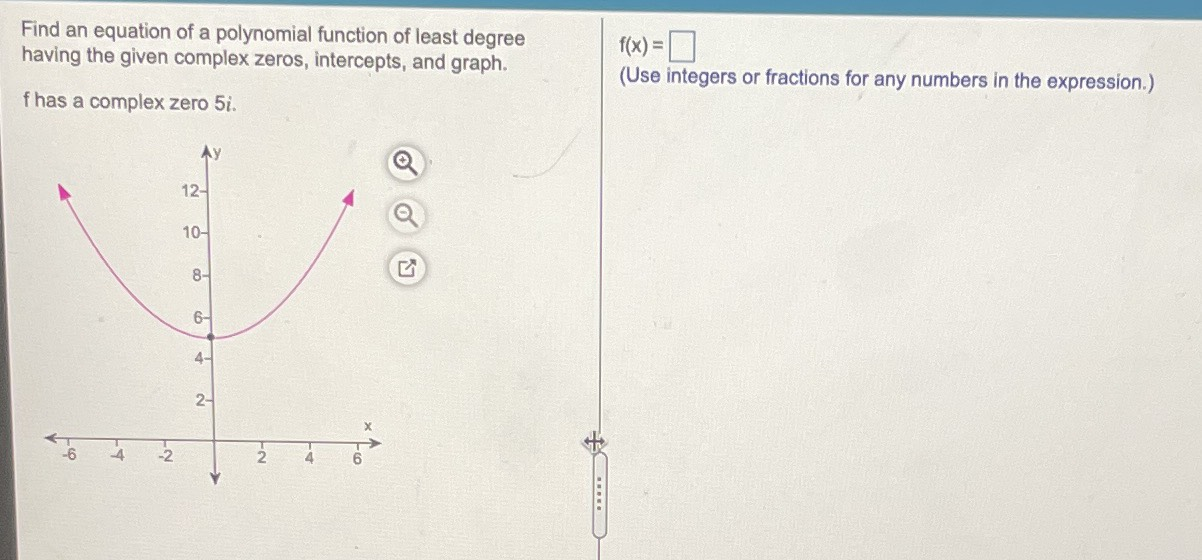Still have math questions?

Algebra
QuestionFind an equation of a polynomial function of least degree having the given complex zeros, intercepts, and graph. $$\mathrm{f}$$ has a complex zero $$5i$$$$f(x)=?$$.

$$f(x)=\frac{1}{5}(x^2+25)$$